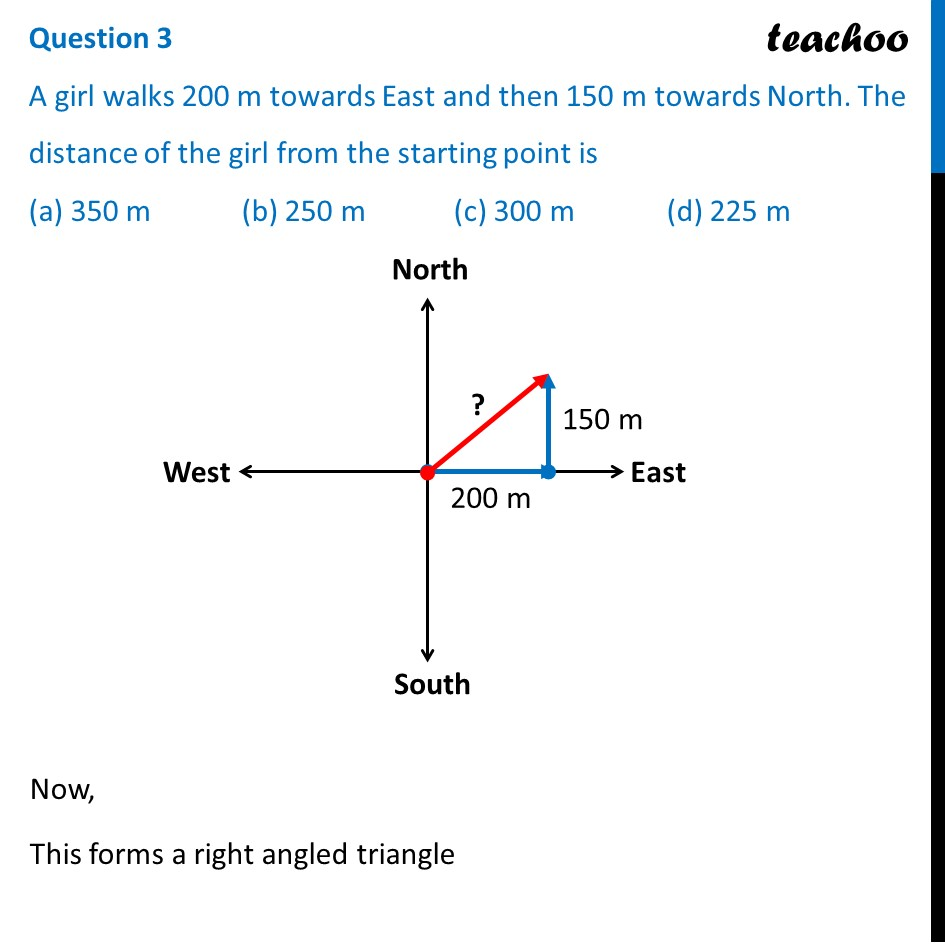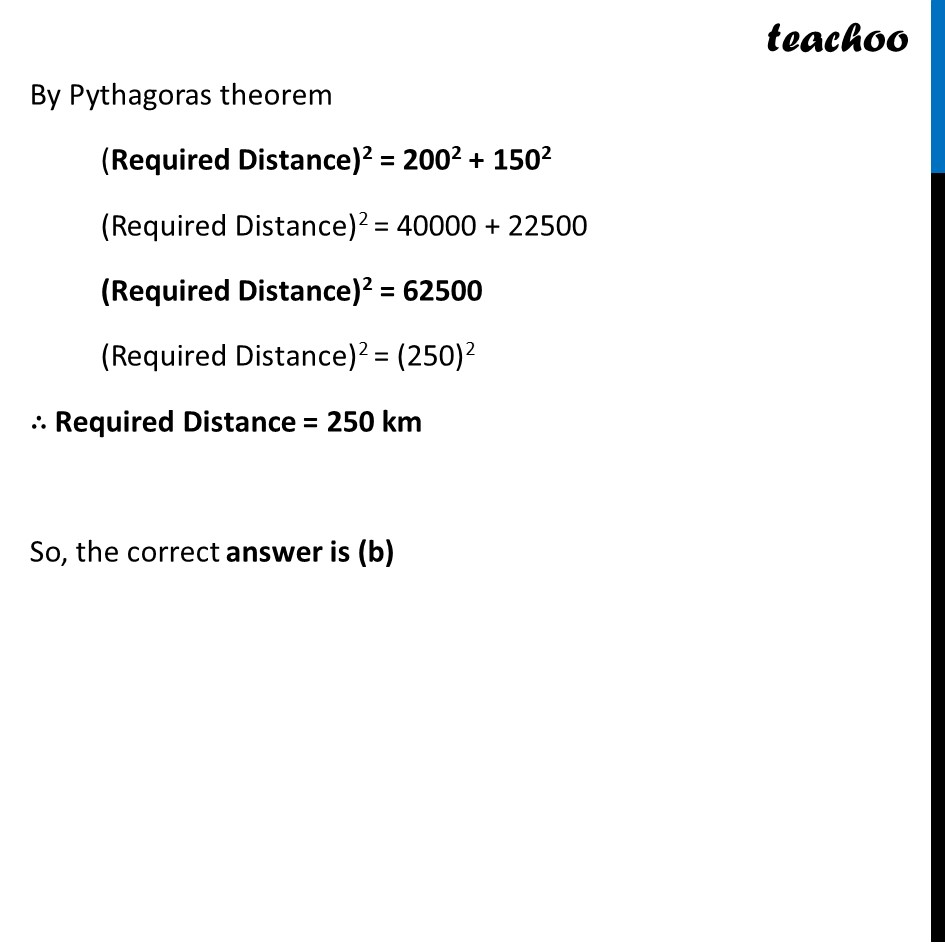## (a) 350 m   (b) 250 m   (c) 300 m   (d) 225 m1. Class 10
2. Solutions of Sample Papers for Class 10 Boards
3. CBSE Class 10 Sample Paper for 2022 Boards - Maths Standard [MCQ]

Transcript

Question 3 A girl walks 200 m towards East and then 150 m towards North. The distance of the girl from the starting point is (a) 350 m (b) 250 m (c) 300 m (d) 225 m By Pythagoras theorem (Required Distance)2 = 2002 + 1502 (Required Distance)2 = 40000 + 22500 (Required Distance)2 = 62500 (Required Distance)2 = (250)2 ∴ Required Distance = 250 km So, the correct answer is (b)